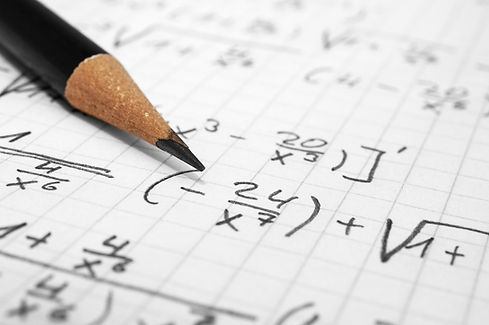Importance of Learning Vedic Maths

Vedic mathematics is a system of mental calculation developed by Shri Bharati Krishna Tirthaji which he claimed he had based on a lost appendix of Atharvaveda, an ancient text of the Indian teachings called Veda. It has some similarities to the Trachtenberg system in that it speeds up some arithmetic calculations. It claims to have applications to more advanced mathematics, such as calculus and linear algebra. The system was first published in the book Vedic Mathematics in 1965.## Level 1, Level 2, Level 3 & Level 4

This list of sutras is taken from the book Vedic Mathematics, which includes a full list of the sixteen Sutras in Sanskrit, but in some cases a translation of the Sanskrit is not given in the text and comes from elsewhere.

## Vedic Maths Course Contents## LEVEL 1 A

• Complements

• Rapid Decimal Addition

• Rapid Multiplication

• Multiplication Tables

• Multiplication of power of 5, 25, 125

• The sum of last digit being 10 and the first digit being the same

• The sum of first digit being 10 and the last digit being the same

• Rapid Division

• Special Method: Division by 5, 25, 125

• VINCULUM

• VINCULATE UNIT'S PLACE, 10's place , 100's place

• Activities## LEVEL 1 B

• Rapid Decimal Addition

• Rapid Subtraction

• Rapid Decimal Subtraction

• Rapid Multiplication

• Multiplication Tables

• Multiplication of power of 5, 25, 125

• The sum of last digit being 10 and the first digit being the same

• The sum of first digit being 10 and the last digit being the same

• Rapid Division

• Special Method: Division by 5, 25, 125

• VINCULUM

• VINCULATE UNIT'S PLACE, 10's place , 100's place

• Activities## LEVEL 2• # Activities

• Rapid Addition - Higher Rows

• Rapid Decimal Addition - Higher Rows

• Rapid Subtraction - Higher Rows

• Multiplication by 11

• Multiplication by 12, 13, .... 19

• Multiplication by 21, 31, .... 91

• Multiplication of numbers nearest to base numbers

• Division of numbers nearest to base numbers (Complementary Method)

• Division by Duplex numbers

• Division by 21, .... 91

• Activities• # Activities

#### Vedic Maths Course Benefits

• It helps a person to solve mathematical problems 10-15 times faster

• It helps in Intelligent Guessing (Knowing the answer without actually solving the problem)

• It reduces burden (Need to learn tables up to nine only)

• It is a magical tool to reduce scratch work and finger counting and improve Mental Calculation

• It increases concentration

• It improves confidence

#### Vedic Maths Batch Details

• For students from 6th std.

• 24 Sessions, 1 hour per session, twice a Week

• Duration 3 Months each level

• Learn online

• Vedic Maths Certificate

• Course Instructor : Holding a degree from "Guru Vedic Maths, Pune"

#### Vedic Maths Books Details

• Level 1 (A & B) - Rs.300 /-

• Level 2              - Rs.150 /-

• Level 3              - Rs.150 /-

• Level 4              - Rs.150 /-

Additional courier charges applicable.

Delivery within 4-5 working days depend on location.